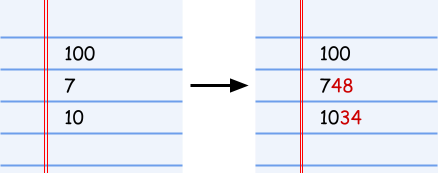시간 제한 메모리 제한 제출 정답 맞은 사람 정답 비율
10 초 (추가 시간 없음) 1024 MB (추가 메모리 없음) 13 10 10 76.923%

## 문제

We have a list of integers X1, X2, …, XN. We would like them to be in strictly increasing order, but unfortunately, we cannot reorder them. This means that usual sorting algorithms will not work.

Our only option is to change them by appending digits 0 through 9 to their right (in base 10). For example, if one of the integers is 10, you can turn it into 100 or 109 with a single append operation, or into 1034 with two operations (as seen in the image below).

Given the current list, what is the minimum number of single digit append operations that are necessary for the list to be in strictly increasing order?

For example, if the list is 100,7,10, we can use 4 total operations to make it into a sorted list, as the following image shows.## 입력

The first line of the input gives the number of test cases, T. T test cases follow. Each test case is described in two lines. The first line of a test case contains a single integer N, the number of integers in the list. The second line contains N integers X1, X2, …, XN, the members of the list.

## 출력

For each test case, output one line containing Case #x: y, where x is the test case number (starting from 1) and y is the minimum number of single digit append operations needed for the list to be in strictly increasing order.

• 1 ≤ T ≤ 100.

## Test Set 1 (12점)

• 2 ≤ N ≤ 3.
• 1 ≤ Xi ≤ 100, for all i.

## Test Set 2 (14점)

• 2 ≤ N ≤ 100.
• 1 ≤ Xi ≤ 109, for all i.

## 예제 입력 1

4
3
100 7 10
2
10 10
3
4 19 1
3
1 2 3


## 예제 출력 1

Case #1: 4
Case #2: 1
Case #3: 2
Case #4: 0


## 힌트

In Sample Case #1, the input is the same as in the example given in the problem statement. As the image shows, the list can be turned into a sorted list with 4 operations. Notice that the last two integers need to end up with at least 3 digits (requiring at least 3 append operations in total). If all of the final numbers had exactly three digits, the second would be larger than the third because it starts with a 7 instead of a 1. This means we cannot do it with fewer than 4 operations.

In Sample Case #2, notice that the list needs to be in strictly increasing order, so we have to do at least one operation. In this case, any valid append operation to the second integer works.

In Sample Case #3, we can use two append operations to get the list to 4,19,193.

In Sample Case #4, the given list is already in strictly increasing order, so no operations are necessary.

## 채점 및 기타 정보

• 예제는 채점하지 않는다.